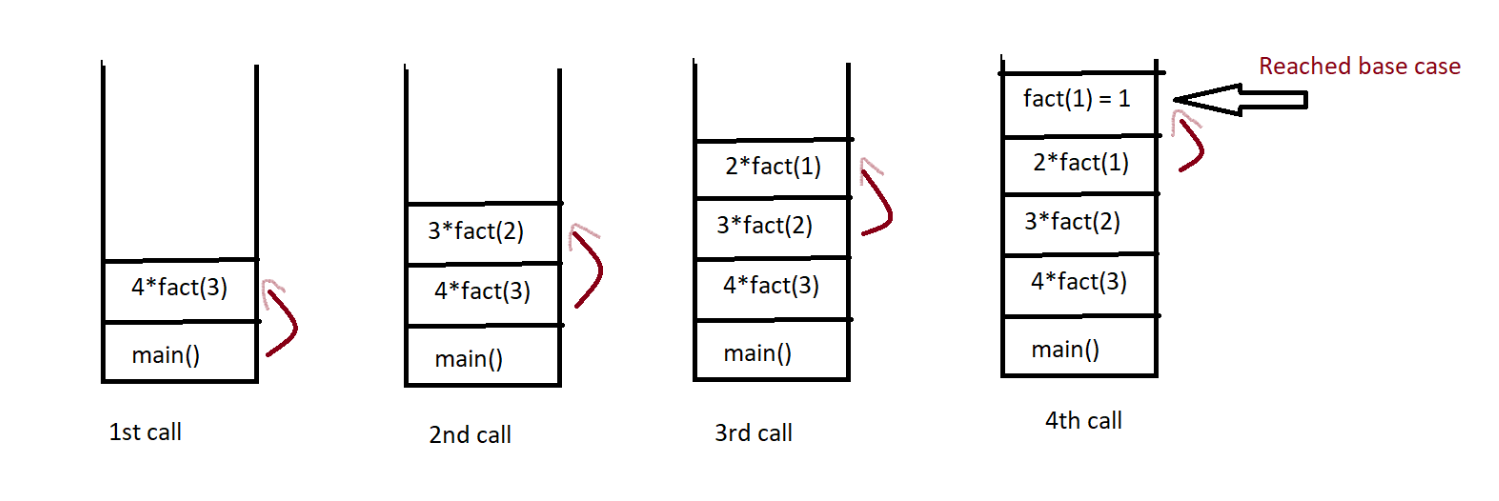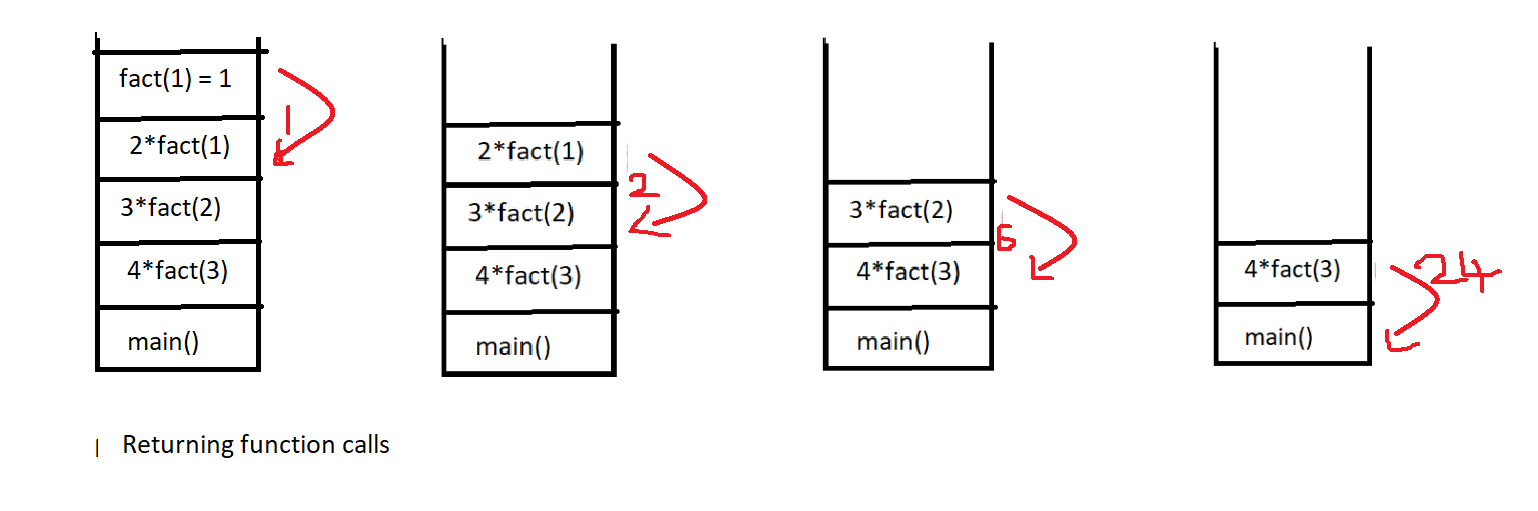# What is Recursion?

What is recursion? A Function calling itself again and again directly or indirectly is called Recursion, and the function which it calls is called a recursive function, it is used in divide and conquer algorithms/techniques.

Let’s see what does the above definition exactly states with an example?

Let’s consider an example of adding the numbers from 1 to 4 using recursion, how to do this?

The mathematical equation for adding numbers would be n+(n-1)+(n-2)+….+1

We will use the above equation to write the code for adding numbers.

• Create a function and pass the value of n(in our case it is 4)
• return the answer which is n+ current value of n -1 + current value of n-1 +…..1

## Java Code

``````public class Recursion {
public static void main(String[] args) {
int n = 4;
System.out.println(rec(n));
}

//function to add the numbers- recursive function

private static int rec(int n) {
return n+(rec(n-1));
}
}
``````

Look at the above example, pass 4 to the rec function

• It will perform 4+(rec(4-1))  // rec(4-1) – calling function again.
Note: Now the current value of n is 3 as rec function took (4-1) which is 3
• It will perform 3+(rec(3-1))  // rec(3-1) – calling function again
Note: Now the current value of n is 2 as rec function took (3-1) which is 2
• It will perform 2+(rec(2-1))  // rec(2-1) – calling function again
Note: Now the current value of n is 1 as rec function took (2-1) which is 1

Now we got the answer which is 4+3+2+1=10, but wait if you observe the above code it will not give the correct answer because it is not stopping the function calling when it reaches 1, it is still calling the same function for values less than 1 also, so here to get the desired output we need to stop that function calling (Also known as BASE CASE) when it reaches to 1, so we will add a condition that if the current value of n becomes 1 then simple stop calling the function and start giving the answer.

### What happens when a recursive call is made:

When the recursive calls are made these calls will be added into a STACK, and will get deleted from the stack once the function starts returning.

### Base Case:

A very important point to note in recursion is the base case or stopping condition of recursive calls, if we don’t give a base case then as you saw in the above example so many recursive calls will be made, memory gets wasted and we will not get desired output. So the base condition is very crucial for recursive code.

### Stack Overflow!

Think what happens when there is no base case?

Without a base case in recursion, the stack will get filled excessively with recursive calls, once the stack memory exceeds the space allocated by JVM then it will lead to a stack overflow error.

Stack overflow error is a runtime error.

There are two types of recursion direct and indirect:

• Direct recursion: Function calling the same function. A function func calls the same function func then it is referred as Direct recursion:
``` //Example of direct recursion
int direct_Func()
{
//code….
......

direct_Func();

}
```
• Indirect recursion: Functions calling another functions again and again. A function func1 is called indirect recursion if this func1 calls a new function func2.
```//Example of Indirect recursion
int indirect_func1()
{
...
...
int indirect_func2();

}
int indirect_func2()
{
...
...
int indirect_func1();

}
```

### Advantages of recursion:

1. Code will be short and clean as we just need to define the base case and the recursive case.
2. To solve such problems which are naturally recursive such as the tower of Hanoi.

### Disadvantages of recursion:

1. Recursive functions are slower than iterative programs.
2. More complex to analyze the code.
3. Not efficient in terms of space, as the stack is required.
4. Not efficient in terms of time complexity too.

Let’s look how the stack calls are actually made and returned with an example:

Consider the question: Print factorial of a number using recursion.

Code:

## Java Code

``````public class Recursion {
public static void main(String[] args) {
int n = 4;
System.out.println(fact(n));
}

//function to find factorial
private static int fact(int n) {

//base case
if (n<=1) return n;

return n*(fact(n-1));
}
}
``````

### Stack calls:Special thanks to Sai bargav Nellepalli for contributing to this article on takeUforward. If you also wish to share your knowledge with the takeUforward fam, please check out this article